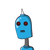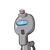# Use Euclid’s division lemma to show that the cube. of any positive integer is of the form 9m,9m+1 or 9m+8​

Use Euclid’s division lemma to show that the cube. of any positive integer is of the form 9m,9m+1 or 9m+8​

### 2 thoughts on “Use Euclid’s division lemma to show that the cube. of any positive integer is of the form 9m,9m+1 or 9m+8​”

1.Let us consider a and b where a be any positive number and b is equal to 3.

According to Euclid’s Division Lemma

a = bq + r

where r is greater than or equal to zero and less than b (0 ≤ r < b)

a = 3q + r

so r is an integer greater than or equal to 0 and less than 3.

Hence r can be either 0, 1 or 2.

Case 1: When r = 0, the equation becomes

a = 3q

Cubing both the sides

a3 = (3q)3

a3 = 27 q3

a3 = 9 (3q3)

a3 = 9m

where m = 3q3

Case 2: When r = 1, the equation becomes

a = 3q + 1

Cubing both the sides

a3 = (3q + 1)3

a3 = (3q)3 + 13 + 3 × 3q × 1(3q + 1)

a3 = 27q3 + 1 + 9q × (3q + 1)

a3 = 27q3 + 1 + 27q2 + 9q

a3 = 27q3 + 27q2 + 9q + 1

a3 = 9 ( 3q3 + 3q2 + q) + 1

a3 = 9m + 1

Where m = ( 3q3 + 3q2 + q)

Case 3: When r = 2, the equation becomes

a = 3q + 2

Cubing both the sides

a3 = (3q + 2)3

a3 = (3q)3 + 23 + 3 × 3q × 2 (3q + 1)

a3 = 27q3 + 8 + 54q2 + 36q

a3 = 27q3 + 54q2 + 36q + 8

a3 = 9 (3q3 + 6q2 + 4q) + 8

a3 = 9m + 8

Where m = (3q3 + 6q2 + 4q)therefore a can be any of the form 9m or 9m + 1 or, 9m + 8.

[tex]\huge{\tt{\red{S}\green{H}\purple{I}\pink{N}\blue{C}\orange{H}\red{A} \green{N} \: \pink{✿༉}}}[/tex]

2.## ❥คɴᎦᴡєя

Let us consider a and b where a be any positive number and b is equal to 3.

According to Euclid’s Division Lemma

a = bq + r

So r is an integer greater than or equal to 0 and less than 3.

Hence r can be either 0, 1 or 2.

Case 1: When r = 0, the equation becomes

a = 3q

Cubing both the sides

a3 = (3q)3

a3 = 27 q3

a3 = 9 (3q3)

a3 = 9m

where m = 3q3

Case 2: When r = 1, the equation becomes

a = 3q + 1

Cubing both the sides

a3 = (3q + 1)3

a3 = (3q)3 + 13 + 3 × 3q × 1(3q + 1)

a3 = 27q3 + 1 + 9q × (3q + 1)

a3 = 27q3 + 1 + 27q2 + 9q

a3 = 27q3 + 27q2 + 9q + 1

a3 = 9 ( 3q3 + 3q2 + q) + 1

a3 = 9m + 1

Where m = ( 3q3 + 3q2 + q)

Case 3: When r = 2, the equation becomes

a = 3q + 2

Cubing both the sides

a3 = (3q + 2)3

a3 = (3q)3 + 23 + 3 × 3q × 2 (3q + 1)

a3 = 27q3 + 8 + 54q2 + 36q

a3 = 27q3 + 54q2 + 36q + 8

a3 = 9 (3q3 + 6q2 + 4q) + 8

a3 = 9m + 8

Where m = (3q3 + 6q2 + 4q)therefore a can be any of the form 9m or 9m + 1 or, 9m + 8.